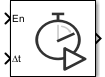# Hit Scheduler

Schedule major time steps for variable-step solver

Since R2022b

•Libraries:

## Description

Use the Hit Scheduler block to schedule major time steps for a variable-step solver during simulation. With the Hit Scheduler block, you can implement dynamic solver hit event scheduling based on the behavior of your model during simulation.

To schedule a major time step, you provide the block two inputs:

• En — The enable input controls when the block schedules a time step. The block schedules a time step when the input is logical `true`.

• Δt — The time interval input specifies when the scheduled time step occurs. The Hit Scheduler block calculates the time hit to schedule, in seconds, as the sum of the current simulation time and the time interval input.

When the simulation reaches the scheduled time hit, the simulation takes a major time step and the block output updates. You can configure the block to produce either a signal output or a function-call output using the Output type parameter.

You can use the Hit Scheduler block to schedule multiple future time steps. The block stores the future time steps in a queue you can configure using the Initial buffer size and Use fixed buffer size parameters.

### Vector Signal Inputs and Outputs

When you configure the block to produce a signal output, you can use a single block to schedule time hits based on multiple signals in the model by using vector inputs. Each element in the En input vector indicates when the block should schedule a time hit based on the same element in the Δt vector. The block output signal has the same dimensions as the input signals. When the simulation takes a scheduled step, the element in the output vector that corresponds to the input vector element that scheduled the time step changes from logical `false` to logical `true`.

## Ports

### Input

expand all

When the enable input value is logical `true`, the block schedules a simulation time step.

When you set Output type to `Signal`, the input can be a scalar or a vector. When the En input is a vector, the Δt input must be a vector with the same dimensions.

When you set Output type to ```Function call```, the input must be a scalar.

Data Types: `Boolean`

The block calculates the time step to schedule, in seconds, as the sum of the current simulation time and the current value of the time interval input.

When you set Output type to `Signal`, the input can be a scalar or a vector. When the Δt input is a vector, the En input must be a vector with the same dimensions.

When you set Output type to ```Function call```, the input must be a scalar.

Data Types: `double`

### Output

expand all

The block output value indicates when the simulation takes a time step the block scheduled. You can configure the block to produce a signal or a function-call event using the Output type parameter. The value of the block output depends on the type of output and the dimensions of the input signal.

• When you set Output type to `Signal` and the input signal is a scalar, the block output becomes logical `true` when the simulation takes a scheduled time step.

• When you set Output type to `Signal` and the input signal is a vector, the element in the output vector that corresponds to the input vector element that scheduled the time step changes from logical `false` to logical `true`.

• When you set Output type to `Function-Call`, the input must be scalar, and the block generates a function-call event when the simulation takes a scheduled time step.

Because the Hit Scheduler block explicitly schedules solver time hits that affect when the block executes, the output has variable sample time for both output types. For more information about variable sample time, see Types of Sample Time.

Data Types: `Boolean`

## Parameters

expand all

The block output indicates when the simulation takes a time step that the block scheduled. You can configure the block to produce a Boolean signal or a function-call event.

• `Signal` — Block output becomes logical `true` when the simulation takes a scheduled time step. Scalar and vector input and output signals are supported.

• `Function Call` — Block generates a function-call event when the simulation takes a scheduled time step. Only scalar input signals are supported.

#### Programmatic Use

 Block Parameter: `HitSchedulerOutputType` Type: string | character vector Values: `'Signal'` | `'Function-Call'` Default: `'Signal'`

Specify the size of the buffer that stores scheduled time steps as a positive, scalar integer value.

By default, the buffer size is dynamic so that the software can increase the size if the buffer fills during simulation. When you select Use fixed buffer size, the Initial buffer size parameter value specifies the fixed size for the buffer. If the buffer overflows during simulation, the Hit Scheduler block overwrites scheduled time hits on a first-in, first-out basis.

#### Programmatic Use

 Block Parameter: `InitialBufferSize` Type: string | character vector Values: positive, whole, numeric scalar Default: `'256'`

When you select this option, the block uses a fixed buffer size, and the Initial buffer size parameter specifies the size of the buffer. If the buffer overflows during simulation, the Hit Scheduler block overwrites scheduled time hits on a first-in, first-out basis. By default, the buffer size is dynamic so that the software can adjust the size during simulation as needed.

#### Programmatic Use

 Block Parameter: `FixedBuffer` Type: string | character vector Values: `'on'` | `'off'` Default: `'off'`

## Block Characteristics

 Data Types `Boolean` | `double` Direct Feedthrough `yes` Multidimensional Signals `no` Variable-Size Signals `no` Zero-Crossing Detection `no`

## Version History

Introduced in R2022b